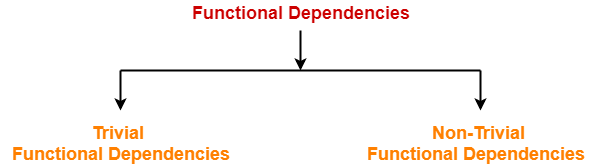Functional Dependency-

 In any relation, a functional dependency α → β holds if-Two tuples having same value of attribute α also have same value for attribute β.

Mathematically,

If α and β are the two sets of attributes in a relational table R where-

• α ⊆ R
• β ⊆ R

Then, for a functional dependency to exist from α to β,

If t1[α] = t2[α], then t1[β] = t2[β]

 α β t1[α] t1[β] t2[α] t2[β] ……. …….

 fd : α → β

Types Of Functional Dependencies-

There are two types of functional dependencies-1. Trivial Functional Dependencies
2. Non-trivial Functional Dependencies

1. Trivial Functional Dependencies-

• A functional dependency X → Y is said to be trivial if and only if Y ⊆ X.
• Thus, if RHS of a functional dependency is a subset of LHS, then it is called as a trivial functional dependency.

Examples-

The examples of trivial functional dependencies are-

• AB → A
• AB → B
• AB → AB

2. Non-Trivial Functional Dependencies-

• A functional dependency X → Y is said to be non-trivial if and only if Y ⊄ X.
• Thus, if there exists at least one attribute in the RHS of a functional dependency that is not a part of LHS, then it is called as a non-trivial functional dependency.

Examples-

The examples of non-trivial functional dependencies are-

• AB → BC
• AB → CD

Inference Rules-

Reflexivity-

If B is a subset of A, then A → B always holds.

Transitivity-

If A → B and B → C, then A → C always holds.

Augmentation-

If A → B, then AC → BC always holds.

Decomposition-

If A → BC, then A → B and A → C always holds.

Composition-

If A → B and C → D, then AC → BD always holds.

If A → B and A → C, then A → BC always holds.

Rules for Functional Dependency-

Rule-01:

A functional dependency X → Y will always hold if all the values of X are unique (different) irrespective of the values of Y.

Example-

Consider the following table-

 A B C D E 5 4 3 2 2 8 5 3 2 1 1 9 3 3 5 4 7 3 3 8

The following functional dependencies will always hold since all the values of attribute ‘A’ are unique-

• A → B
• A → BC
• A → CD
• A → BCD
• A → DE
• A → BCDE

In general, we can say following functional dependency will always hold-

 A → Any combination of attributes A, B, C, D, E

Similar will be the case for attributes B and E.

Rule-02:

A functional dependency X → Y will always hold if all the values of Y are same irrespective of the values of X.

Example-

Consider the following table-

 A B C D E 5 4 3 2 2 8 5 3 2 1 1 9 3 3 5 4 7 3 3 8

The following functional dependencies will always hold since all the values of attribute ‘C’ are same-

• A → C
• AB → C
• ABDE → C
• DE → C
• AE → C

In general, we can say following functional dependency will always hold true-

 Any combination of attributes A, B, C, D, E → C

Combining Rule-01 and Rule-02 we can say-

 In general, a functional dependency α → β always holds-If either all values of α are unique or if all values of β are same or both.

Rule-03:

For a functional dependency X → Y to hold, if two tuples in the table agree on the value of attribute X, then they must also agree on the value of attribute Y.

Rule-04:

For a functional dependency X → Y, violation will occur only when for two or more same values of X, the corresponding Y values are different.

Next Article- Equivalence of Functional Dependencies

Get more notes and other study material of Database Management System (DBMS).

Watch video lectures by visiting our YouTube channel LearnVidFun.